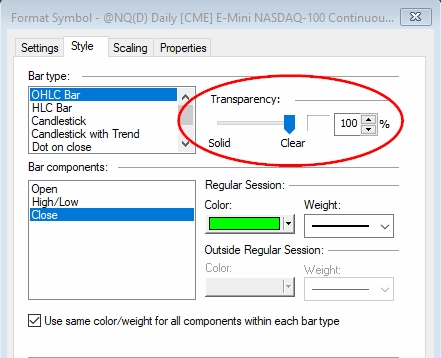This is a simple indicator but it does involve some semi-advanced topics.  Just to let you know I am working on the third book in the Easing Into EasyLanguage series.  If you haven’t check out the first two, you might just want to head over to amazon and check those out.  This topic falls in the spectrum of the ideas that I will be covering in the Advanced Topics edition.  Also to let you know I just published the 2nd Edition of Trend Following Systems: A DIY Project – Batteries Included.  Check this out if you want to learn some Python and also see some pretty cool Trend Following algorithms – I include EasyLanguage too!

The code that follows demonstrates how to shade between plots and adjust gradient in terms of the RSI reading.  I compiled this with MultiCharts, so I assume it will work there too – just let me know if it doesnt.  I found this code somewhere on the web when researching shading.  If I knew the original author I would definitely give full credit.   The code is rather simple, setting up the chart is just slightly more difficult.  The Keltner Channel was used to define the shading boundaries.  You could have just as easily used Bollinger Bands or anything that provided a range around the market.  Here’s the code.

``inputs:  KeltnerLength( 90 ), KeltnerWid( 5 ), RSILength( 14 ), overbought( 70 ), oversold( 30 ); var:  Avg( 0 ), Shift( 0 ), LowerBand( 0 ), UpperBand( 0 ), MyRSI( 0 ) ;  // Keltner  Avg = AverageFC( c, KeltnerLength ) ; Shift = KeltnerWid * AvgTrueRange( Keltnerlength ) ; UpperBand = Avg + Shift ; LowerBand = Avg - Shift ;  Plot11( UpperBand, "UpperBand" ) ; Plot12( LowerBand, "LowerBand" ) ; Plot13( Avg, "MidLine" ) ;  // RSI  MyRSI = xaverage(RSI( c, RSILength ), 7) ;  var: projrsi(0); // Get projected RSI in terms of the Upper and Lower Bandsprojrsi = Avg + .01 * (UpperBand - LowerBand) * (MyRSI - 50) * 2.5; if false then plot14( projrsi, "RSI" );  // Gradient background  var: barspacing( getappinfo( aibarspacing ) ); var: gradcolr(0); // Remember how to use the IFF function?gradcolr = iff( MyRSI > 50, GradientColor( projrsi, Avg, UpperBand, black, red),  	GradientColor(projrsi, LowerBand, Avg, green, black) );  plot91( UpperBand, "ugrad", gradcolr, default, barspacing); plot92( LowerBand, "lgrad");  // Show Bar - increase transparency of data to 100% so// shading does not overlap the bar charts plot4( c, "c"); plot5( h, "h"); plot6( l, "l");``

That is a little bit of code that does a lot of work.  Here are the key lines and their explanations.

projrsi = Avg + .01 * (UpperBand – LowerBand) * (MyRSI – 50) * 2.5;

Remember the RSI outputs values between 0 and 100 – oscillates.  Assume RSI is in oversold territory at 24.

UpperBand = 16273 and LowerBand = 15023 and Avg = 15648

Let’s do the math:

1. projrsi = 15468 + 0.01 * (16273 – 15023) * (24 – 50) * 2.5
2. projrsi = 15468 + 0.01 * 1250  * – 26 * 2.5
3. projrsi = 15468 + 12.5 * -65
4. projrsi = 15468 – 165
5. projrsi = 15308

Basically all this math is doing is keeping the RSI reading within the bounds of the Keltner Upper and Lower Channels.  You want a high RSI reading to be near the Upper Channel and a low RSI reading to be near the Lower Channel.   You can change up the formula to make more sense.

projrsi = Avg + (MyRSI – 50)/100 * (UpperBand – LowerBand) * 2.5

I have worked with computer graphics for many years and this is really a very neat formula.  The generic formula to constrain a value within a boundary is;

projrsi = LowerBand + (MyRSI / 100) * (UpperBand – LowerBand)

Here you take the LowerBand and add the percentage of the MyRSI/100 times the range.  This works too.  But the original formula scales or intensifies the RSI reading so you get much wider gradient spectrum.  The AVG is used as the center of gravity and the RSI is converted in terms of the middle 50 line.  A positive number, anything > 50, is then scaled higher in the range and a negative number, anything < 50 is scaled lower in the range.  In other words it makes a prettier and more informative picture.

The other important line in the code is

gradcolr = iff( MyRSI > 50, GradientColor( projrsi, Avg, UpperBand, black, red),
GradientColor(projrsi, LowerBand, Avg, green, black) );

This code uses the IFF function which basically replicates this

If MyRSI > 50 then

else

GradientColor( dValue, dMin, dMax, nFromColor, nToColor )

Return

Returns a specific color from a user defined gradient color range, such as Blue to White

Inputs:

• dValue = value being passed-in that is within the specified Min/Max range of values
• dMin = Starting value for the gradient range, where the nFromColor is displayed
• dMax = Ending value for the gradient range, where the nToColor is displayed
•  nFromColor = Starting color of the gradient
• nToColor = Ending color of the gradient

Since the gradient shading will cover up your bars you will need to plot the bars as well.

## Chart SetUp